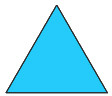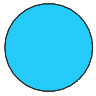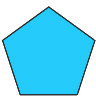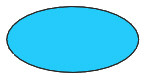# Area of ​​geometric Figures: Definition, Formulas with ExamplesThe area of ​​a geometric figure is a measure that quantifies the amount of surface or space contained within the boundaries of that figure in a plane. In other words, area tells us how much space a shape occupies on a two-dimensional surface, such as a piece of paper, a computer screen, or any plane.

The area may be expressed in square mm, such as square centimeters (cm²), square meters (m²), or any other suitable unit of area.

The method for calculating the area of ​​a geometric figure depends on the specific shape of the figure. Each type of figure has its own mathematical formula to determine its area.

Below I attach the calculation of the area of ​​the 10 most important two-dimensional geometric figures along with their description, formula and a numerical example:

## Area of ​​a Rectangle: Formula and Example

A rectangle is a quadrilateral with four right angles. The area of ​​a rectangle is calculated by multiplying its length by its width.

Formula: Area (A) = Length (l) x Width (w)

### Example

If we have a rectangle with a length of 8 mm and a width of 5 mm, then the area would be: A = 8 mm x 5 mm = 40 square mm.

## Area of ​​a Triangle: Formula and ExampleA triangle is a figure with three sides and three angles. The area of ​​a triangle is calculated by multiplying the length of its base by its height and dividing the result by 2.

Formula: Area (A) = (Base x Height) / 2

### Example

If we have a triangle with a base of 6 mm and a height of 4 mm, then the area would be: A = (6 mm x 4 mm) / 2 = 12 mm square.

## Area of ​​a Circle: Formula and ExampleA circle is a round figure with all points on its perimeter at the same distance from the center. The area of ​​a circle is calculated by multiplying π (pi) by the square of the radius.

Formula: Area (A) = π x Radius (r) squared (r²)

### Example

If we have a circle with a radius of 3 mm, then the area would be: A = π x (3 mm)² = 9π square mm (approximately 28.27 square mm).

## Area of ​​a Square: Formula and ExampleA square is a rectangle with all sides of equal length. The area of ​​a square is calculated by squaring the length of one of its sides.

Formula: Area (A) = Side (s) squared (s²)

### Example

If we have a square with a side of 5 mm, then the area would be: A = (5 mm)² = 25 mm square.

## Area of ​​a Rhombus: Formula and ExampleA rhombus is a quadrilateral with all sides of equal length but non-right angles. The area of ​​a rhombus is calculated by multiplying the length of the major diagonal by the minor diagonal and dividing the result by 2.

Formula: Area (A) = (Major Diagonal x Minor Diagonal) / 2

### Example

If we have a rhombus with diagonals of 6 mm and 8 mm, then the area would be: A = (6 mm x 8 mm) / 2 = 24 mm square.

## Area of ​​a Parallelogram: Formula and Example

A parallelogram is a quadrilateral with opposite parallel sides. The area of ​​a parallelogram is calculated by multiplying the length of its base by its height.

Formula: Area (A) = Base x Height

### Example

If we have a parallelogram with a base of 7 mm and a height of 4 mm, then the area would be: A = 7 mm x 4 mm = 28 square mm.

## Area of ​​a Trapezoid: Formula and Example

A trapezoid is a quadrilateral with two parallel sides and two non-parallel sides. The area of ​​a trapezoid is calculated by adding the lengths of the largest and shortest bases, multiplying the result by the height, and dividing by 2.

Formula: Area (A) = ((Major Base + Minor Base) x Height) / 2

### Example

If we have a trapezoid with bases of 5 mm and 9 mm, and a height of 6 mm, then the area would be: A = ((5 mm + 9 mm) x 6 mm) / 2 = 42 square mm.

## Area of ​​a Regular Pentagon: Formula and ExampleA regular pentagon is a polygon with five sides and equal angles. The area of ​​a regular pentagon is calculated by multiplying the perimeter by the apothem (the distance from the center to the midpoint of a side) and dividing the result by 2.

Formula: Area (A) = (Perimeter x Apothem) / 2

### Example

If we have a regular pentagon with a perimeter of 20 mm and an apothem of 4 mm, then the area would be: A = (20 mm x 4 mm) / 2 = 40 square mm.

## Area of ​​a Regular Hexagon: Formula and ExampleA regular hexagon is a polygon with six sides and equal angles. The area of ​​a regular hexagon is calculated by multiplying the perimeter by the apothem and dividing the result by 2.

Formula: Area (A) = (Perimeter x Apothem) / 2

### Example

If we have a regular hexagon with a perimeter of 24 mm and an apothem of 5 mm, then the area would be: A = (24 mm x 5 mm) / 2 = 60 square mm.

## Area of ​​an Ellipse: Formula and ExampleAn ellipse is a figure similar to a circle, but with axes of different lengths. The area of ​​an ellipse is calculated by multiplying π (pi) by the product of its semimajor and minor axes.

Formula: Area (A) = π x Semi-Major Axis (a) x Semi-Minor Axis (b)

### Example

If we have an ellipse with semi-major axes (a) of 6 mm and semi-minor axis (b) of 4 mm, then the area would be: A = π x 6 mm x 4 mm = 24π square mm (approximately 75.40 square mm).

Author:

Published: September 14, 2023
Last review: September 14, 2023